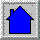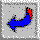# STEPHEN E. MENDES

## PASCAL PROGRAMSThese programs were compiled with Microsoft Pascal compiler, slight changes will be required for Turbo Pascal.List ADT 1
These first 3 programs are the same, except for the list ADT. The program reads an input file of single digit integers, one per line, and puts them into a list L (the ADT). The list is then printed, purged of duplicate elements and printed again. This one uses the ARRAY model.List ADT 2
As above, but this one uses the LINKED LIST model with NO header cell.List ADT 3Hash Storage
This program demonstrates hashing. It accepts the same input as the ones above, but the integers do NOT have to be single digit. It uses the list model 2 (above). You enter the maximum number of Buckets and the program prints a storage map.Traverse Graph using DFS
The input file must completely define the graph.Polynomial Addition
Takes two input files. Each line in an input file contains a single term (the coefficient, followed by a space, then the exponent). the polynomials can be any length, but the terms must be in the same order for both files (ie. least to greatest, or vica versa).Polynomial Multiplication
Takes two input files. Each line in an input file contains a single term (the coefficient, followed by a space, then the exponent). the polynomials can be any length, but the terms must be in the same order for both files (ie. least to greatest, or vica versa).Stack demo
Uses two stacks to evaluate arithmetic expressions. Operators are limited to add and multiply and operands limited to single digit integers.Post order tree traversal
The tree is specified in the input file as: node, space, parent node (on each line).Trace program execution
This program is the same as LIST example 2 above, but now a circular queue is used to trace the execution of the algorithm. the constant maxlength determines the queue size.Priority Queue
Several insertions (events) are added to the queue as the program runs (these are hard coded into the program for simplicity) also serveral events are removed from the queue. The HEAP model is used and the integers added represent the priority of the events, with a lower number meaning HIGHER priority.Quicksort
This is a sort program that sorts an input file of integers.Back to Homepage.Back to PROGRAM page.